## Applied Machine Learning Classification for Decision Making

Making the good decision is the challenge that we often have. So, in this post, we will look at how applied machine learning classification can be used for the process of decision making.

The simple and quick approach to make decision is follow our past experience of similar situations. Usually we use compiling a list of pros and cons, asking someone for help or searching on the web. According to  we have two systems in our brain: logical and intuitive system:

“With every decision you take, every judgement you make, there is a battle in your mind – a battle between intuition and logic.”

“Most of the beliefs or opinions you have come from an automatic response. But then your logical mind invents a reason why you think or believe something.”

Most of the time our intuitive system is working efficiently, taking charge of all the thousands of decisions we make each day. But our intuitive system can be biased in many ways. For example it can be biased toward the latest unsuccessful outcome.

Besides this our memory can not remember a lot of information so we can not use efficiently all our past information and experience. That’s why people created tools like decision matrix that can help to improve decision making. In the next section we will look at techniques that facilitates using our rational decision making.## Decision Matrix

More advanced approach for making decision is score each possible option. In this approach we score each option against some criteria or feature. For example for the candidate product that we need to buy we are looking at price, quality, service and safety features. This approach results in creating decision matrix for analysis of possible options.

Below is an example of decision matrix  for choosing strategy for building some software project. Here we score 4 options based on the time to build and cost. The score is on the scale 0 (worst) – 100 (best). After we score each cell for time and cost rows, we can get sum of scores, rank and then make our choice (see last 3 rows)Decision Matrix Example

There are also other, similar to decision matrix tools: belief decision matrix, Pugh Matrix.
These tools allow do comparison analysis of available options vs features and this enforces our mind logically evaluate and rank as much as possible pros and cons based on some numerical metrics.

However there are some limitations also. Incorrect selection criteria will obviously lead to the wrong conclusion. Poorly defined criteria can have multiple interpretations. For example too low can mean different things. With many rows or columns it becomes labor intensive to fill out the matrix.

## Machine Learning Approach for Making Decision

Machine learning techniques can also help us improve decision making and even solve some of the above limitations. For example with Feature Engineering we can evaluate what criteria are important for decision.

In machine learning making decision can be viewed as assigning or predicting correct label (for example buy, not buy) based on data for the item features. In the field of machine learning or AI this is known as classification problem.

Classification algorithms learn correct decisions from data. Below is the example of training data that we input to machine learning classification algorithm. (Xij represent some numerical values)Classification problem

Our options (decisions) are now represented by class label (most right column), criteria are represented by features. So we now switched columns with rows. Using training data like above we train classifier and then use it to choose the class (make decision) for new data.

There are different classification algorithms such as decision tree, SVM, Naive Bayes, neural network classification. In this post we will look at classification with neural network.
We will use Keras neural network with 2 dense layers.

Per Keras documentation Dense layer implements the operation:
output = activation(dot(input, kernel) + bias)
where activation is the element-wise activation function passed as the activation argument,
kernel is a weights matrix created by the layer,
and bias is a bias vector created by the layer (only applicable if use_bias is True).

So we can see that the dense layer is performing similar math that we were doing in decision matrix.

## Python Source Code for Neural Network Classification Algorithms

Finally, below is the python source code for classification problem. To test this code we will use iris dataset. This dataset has 3 classes and 4 features. Our task here to make the classifier able to assign correct label class.

```# -*- coding: utf-8 -*-

from keras.utils import to_categorical
from sklearn import datasets

iris = datasets.load_iris()

# Create feature matrix
X = iris.data
print (X)

# Create target vector
y = iris.target
y = to_categorical(y, num_classes=3)
print (y)

from sklearn.model_selection import train_test_split

x_train, x_test, y_train, y_test = train_test_split( X, y, test_size=0.33, random_state=42)

print (x_train)
print (y_train)

from keras.models import Sequential
from keras.layers import Dense

model = Sequential()
model.add(Dense(32, input_shape=(4,), activation='relu',  name='L1'))
model.add(Dense(3, activation='softmax', name='L2'))
model.compile(optimizer='rmsprop',
loss='categorical_crossentropy',
metrics=['accuracy'])

print('Model Summary:')
print(model.summary())

model.fit(x_train, y_train, verbose=2, batch_size=10, epochs=100)
output = model.evaluate(x_test, y_test)

print('Final test loss: {:4f}'.format(output))
print('Final test accuracy: {:4f}'.format(output))
```

Below are results of neural network run.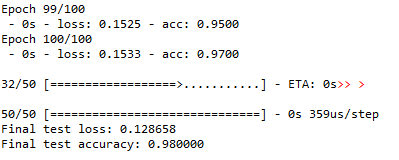Results of neural network run

As we can see our classifier is able to make correct decisions with 98% accuracy.

Thus we investigated different approaches for making decision. We saw how machine learning can be applied to this too. Specifically we looked at neural network classification algorithm for selecting correct label.
I would love to hear what types of decision making tools do you use for making decisions? Also feel free to provide feedback or suggestions.

## Application for Machine Learning for Analyzing Blog Text and Google Analytics Data

In the previous post we looked how to download data from WordPress blog.  So now we can have blog data. We can get also web metrics data from Google Analytics such us the number of views, time on the page. How do we connect post text data with metrics data to see how different topics/keywords correlate with different metrics data? Or may be we want to know what terms contribute to higher time on page or number of views?

Here is the experiment that we can do to check how we can combine blog post text data with web metrics. I downloaded data from blog and saved in the csv file. This is actually same file that was obtained in .In this file time on page from Google Analytics was added manually as additional column. The python program was created. In the program the numeric value in sec is converted in two labels 0 and 1 where 0 is assigned if time less than 120 sec, otherwise 1 is assigned.

``````
Then machine learning was applied as below:
for each label
load the post data that have this label from file
apply TfidfVectorizer
cluster data
save data in dataframe
print dataframe
``````

So the dataframe will show distribution of keywords for groups of posts with different time on page.
This is useful if we are interesting why some posts doing well and some not.

Below is sample output and source code:``````
# -*- coding: utf-8 -*-

from sklearn.cluster import KMeans
from sklearn.feature_extraction.text import TfidfVectorizer
import numpy as np
import pandas as pd

pd.set_option('max_columns', 50)

#only considers the top n words ordered by term frequency
n_features=250
use_idf=True
number_of_runs = 3

import csv
import re

def remove_html_tags(text):
"""Remove html tags from a string"""
clean = re.compile('<.*?>')
return re.sub(clean, '', text)

fn="posts.csv"
labelsY=[0,1]
k=3

exclude_words=['row', 'rows', 'print', 'new', 'value', 'column', 'count', 'page', 'short', 'means', 'newline', 'file', 'results']
columns = ['Low Average Time on Page', 'High Average Time on Page']
index = np.arange(50) # array of numbers for the number of samples
df = pd.DataFrame(columns=columns , index = index)

for z in range(len(labelsY)):

doc_set = []

with open(fn, encoding="utf8" ) as f:
csv_f = csv.reader(f)
for i, row in enumerate(csv_f):
if i > 1 and len(row) > 1 :
include_this = False
if  labelsY[z] ==0:
if (int(row)) < 120 :
include_this=True
if  labelsY[z] ==1:
if (int(row)) >= 120 :
include_this=True

if  include_this:
temp=remove_html_tags(row)
temp=row + " " + temp
temp = re.sub("[^a-zA-Z ]","", temp)

for word in exclude_words:
if word in temp:
temp=temp.replace(word,"")
doc_set.append(temp)

vectorizer = TfidfVectorizer(max_df=0.5, max_features=n_features,
min_df=2, stop_words='english',
use_idf=use_idf)

X = vectorizer.fit_transform(doc_set)
print("n_samples: %d, n_features: %d" % X.shape)

km = KMeans(n_clusters=k, init='k-means++', max_iter=100, n_init=1)
km.fit(X)
order_centroids = km.cluster_centers_.argsort()[:, ::-1]
terms = vectorizer.get_feature_names()
count=0
for i in range(k):
print("Cluster %d:" % i, end='')
for ind in order_centroids[i, :10]:
print(' %s' % terms[ind], end='')
df.set_value(count, columns[z], terms[ind])
count=count+1

print ("\n")
print (df)
``````

References

## Time Series Prediction with Convolutional Neural Networks and Keras

A convolutional neural network (CNNs) is a type of network that has recently
gained popularity due to its success in classification problems (e.g. image recognition
or time series classification) . One of the working examples how to use Keras CNN for time series can be found at this link. This example allows to predict for single time series and multivariate time series.

Running the code from this link, it was noticed that sometimes the prediction error has very high value, may be because optimizer gets stuck in local minimum. (See Fig1. The error is on axis Y and is very high for run 6) So I updated the script to run several times and then remove results with high error. (See Fig2 The Y axis showing small error values). Here is the summary of all changes:

• Created multiple runs that can allow to filter bad results based on error. Training CNN is running 10 times and for each run error data and some other associated data is saved. Error is calculated as square root of sum if squared errors for last 10 predictions during the training.
• Added also plot to see error over multiple runs.
• In the end of script added one plot that showing errors for each run (See Fig1.) , and another plot showing errors only for runs that did not have high error (See Fig2.).
• Added saving keras model to file for each run and then loading it from file for model that showed best results (min error). See  for more information on saving and loading keras models.
• Added ability to load time series from csv file.Error for all runsFig 1.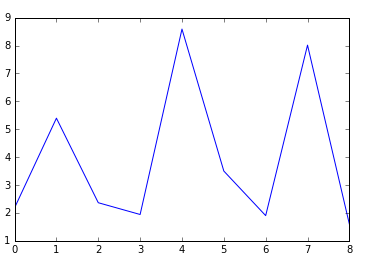Error chart after removing runs with high value errorFig 2.

Below is the full code

``````
#!/usr/bin/env python
"""
This code is based on convolutional neural network model from below link
gist.github.com/jkleint/1d878d0401b28b281eb75016ed29f2ee
"""

from __future__ import print_function, division

import numpy as np
from keras.layers import Convolution1D, Dense, MaxPooling1D, Flatten
from keras.models import Sequential
from keras.models import model_from_json

import matplotlib.pyplot as plt
import csv

__date__ = '2017-06-22'

error_total =[]
result=[]
i=0

def make_timeseries_regressor(window_size, filter_length, nb_input_series=1, nb_outputs=1, nb_filter=4):
""":Return: a Keras Model for predicting the next value in a timeseries given a fixed-size lookback window of previous values.

The model can handle multiple input timeseries (`nb_input_series`) and multiple prediction targets (`nb_outputs`).

:param int window_size: The number of previous timeseries values to use as input features.  Also called lag or lookback.
:param int nb_input_series: The number of input timeseries; 1 for a single timeseries.
The `X` input to ``fit()`` should be an array of shape ``(n_instances, window_size, nb_input_series)``; each instance is
a 2D array of shape ``(window_size, nb_input_series)``.  For example, for `window_size` = 3 and `nb_input_series` = 1 (a
single timeseries), one instance could be ``[, , ]``. See ``make_timeseries_instances()``.
:param int nb_outputs: The output dimension, often equal to the number of inputs.
For each input instance (array with shape ``(window_size, nb_input_series)``), the output is a vector of size `nb_outputs`,
usually the value(s) predicted to come after the last value in that input instance, i.e., the next value
in the sequence. The `y` input to ``fit()`` should be an array of shape ``(n_instances, nb_outputs)``.
:param int filter_length: the size (along the `window_size` dimension) of the sliding window that gets convolved with
each position along each instance. The difference between 1D and 2D convolution is that a 1D filter's "height" is fixed
to the number of input timeseries (its "width" being `filter_length`), and it can only slide along the window
dimension.  This is useful as generally the input timeseries have no spatial/ordinal relationship, so it's not
meaningful to look for patterns that are invariant with respect to subsets of the timeseries.
:param int nb_filter: The number of different filters to learn (roughly, input patterns to recognize).
"""
model = Sequential((
# The first conv layer learns `nb_filter` filters (aka kernels), each of size ``(filter_length, nb_input_series)``.
# Its output will have shape (None, window_size - filter_length + 1, nb_filter), i.e., for each position in
# the input timeseries, the activation of each filter at that position.
Convolution1D(nb_filter=nb_filter, filter_length=filter_length, activation='relu', input_shape=(window_size, nb_input_series)),
MaxPooling1D(),     # Downsample the output of convolution by 2X.
Convolution1D(nb_filter=nb_filter, filter_length=filter_length, activation='relu'),
MaxPooling1D(),
Flatten(),
Dense(nb_outputs, activation='linear'),     # For binary classification, change the activation to 'sigmoid'
))
model.compile(loss='mse', optimizer='adam', metrics=['mae'])
# To perform (binary) classification instead:
# model.compile(loss='binary_crossentropy', optimizer='adam', metrics=['binary_accuracy'])
return model

def make_timeseries_instances(timeseries, window_size):
"""Make input features and prediction targets from a `timeseries` for use in machine learning.

:return: A tuple of `(X, y, q)`.  `X` are the inputs to a predictor, a 3D ndarray with shape
``(timeseries.shape - window_size, window_size, timeseries.shape or 1)``.  For each row of `X`, the
corresponding row of `y` is the next value in the timeseries.  The `q` or query is the last instance, what you would use
to predict a hypothetical next (unprovided) value in the `timeseries`.
:param ndarray timeseries: Either a simple vector, or a matrix of shape ``(timestep, series_num)``, i.e., time is axis 0 (the
row) and the series is axis 1 (the column).
:param int window_size: The number of samples to use as input prediction features (also called the lag or lookback).
"""
timeseries = np.asarray(timeseries)
assert 0 < window_size < timeseries.shape
X = np.atleast_3d(np.array([timeseries[start:start + window_size] for start in range(0, timeseries.shape - window_size)]))
y = timeseries[window_size:]
q = np.atleast_3d([timeseries[-window_size:]])
return X, y, q

def evaluate_timeseries(timeseries, window_size):
"""Create a 1D CNN regressor to predict the next value in a `timeseries` using the preceding `window_size` elements
as input features and evaluate its performance.

:param ndarray timeseries: Timeseries data with time increasing down the rows (the leading dimension/axis).
:param int window_size: The number of previous timeseries values to use to predict the next.
"""
filter_length = 5
nb_filter = 4
timeseries = np.atleast_2d(timeseries)
if timeseries.shape == 1:
timeseries = timeseries.T       # Convert 1D vectors to 2D column vectors

nb_samples, nb_series = timeseries.shape
print('\n\nTimeseries ({} samples by {} series):\n'.format(nb_samples, nb_series), timeseries)
model = make_timeseries_regressor(window_size=window_size, filter_length=filter_length, nb_input_series=nb_series, nb_outputs=nb_series, nb_filter=nb_filter)
print('\n\nModel with input size {}, output size {}, {} conv filters of length {}'.format(model.input_shape, model.output_shape, nb_filter, filter_length))
model.summary()

error=[]

X, y, q = make_timeseries_instances(timeseries, window_size)
print('\n\nInput features:', X, '\n\nOutput labels:', y, '\n\nQuery vector:', q, sep='\n')
test_size = int(0.01 * nb_samples)           # In real life you'd want to use 0.2 - 0.5
X_train, X_test, y_train, y_test = X[:-test_size], X[-test_size:], y[:-test_size], y[-test_size:]
model.fit(X_train, y_train, nb_epoch=25, batch_size=2, validation_data=(X_test, y_test))

# serialize model to JSON
model_json = model.to_json()
with open("model"+str(i)+".json", "w") as json_file:
json_file.write(model_json)
# serialize weights to HDF5
model.save_weights("model"+str(i)+".h5")
print("Saved model to disk")
global i
i=i+1

pred = model.predict(X_test)
print('\n\nactual', 'predicted', sep='\t')
error_curr=0
for actual, predicted in zip(y_test, pred.squeeze()):
print(actual.squeeze(), predicted, sep='\t')
tmp = actual-predicted
sum_squared = np.dot(tmp.T , tmp)
error.append ( np.sqrt(sum_squared) )
error_curr=error_curr+ np.sqrt(sum_squared)
print('next', model.predict(q).squeeze(), sep='\t')
result.append  (model.predict(q).squeeze())
error_total.append (error_curr)
print (error)

def read_file(fn):
'''
Reads the CSV file
-----
RETURNS:
A matrix with the file contents
'''

vals = []
with open(fn, 'r') as csvfile:
tsdata = csv.reader(csvfile, delimiter=',')
for row in tsdata:
vals.append(row)

# removing title row
vals = vals[1:]
y = np.array(vals).astype(np.float)
return y

def main():
"""Prepare input data, build model, eval uate."""
np.set_printoptions(threshold=25)
ts_length = 1000
window_size = 50
number_of_runs=10
error_max=200

print('\nSimple single timeseries vector prediction')
timeseries = np.arange(ts_length)                   # The timeseries f(t) = t
# enable below line to run this time series
#evaluate_timeseries(timeseries, window_size)

print('\nMultiple-input, multiple-output prediction')
timeseries = np.array([np.arange(ts_length), -np.arange(ts_length)]).T      # The timeseries f(t) = [t, -t]
# enable below line to run this time series
##evaluate_timeseries(timeseries, window_size)

print('\nMultiple-input, multiple-output prediction')
timeseries = np.array([np.arange(ts_length), -np.arange(ts_length), 2000-np.arange(ts_length)]).T      # The timeseries f(t) = [t, -t]
# enable below line to run this time series
#evaluate_timeseries(timeseries, window_size)

timeseries = read_file('ts_input.csv')
print (timeseries)

for i in range(number_of_runs):
evaluate_timeseries(timeseries, window_size)

error_total_new=[]
for i in range(number_of_runs):
if (error_total[i] < error_max):
error_total_new.append (error_total[i])

plt.plot(error_total)
plt.show()
print (result)

plt.plot(error_total_new)
plt.show()
print (result)

best_model=np.asarray(error_total).argmin(axis=0)
print ("best_model="+str(best_model))

json_file = open('model'+str(best_model)+'.json', 'r')
loaded_model_json = json_file.read()
json_file.close()
loaded_model = model_from_json(loaded_model_json)

# load weights into new model
loaded_model.load_weights("model"+str(best_model)+".h5")
print("Loaded model from disk")

if __name__ == '__main__':
main()

``````

## Forecasting Time Series Data with Convolutional Neural Networks

Convolutional neural networks(CNN) is increasingly important concept in computer science and finds more and more applications in different fields. Many posts on the web are about applying convolutional neural networks for image classification as CNN is very useful type of neural networks for image classification. But convolutional neural networks can also be used for applications other than images, such as time series prediction. This post is reviewing existing papers and web resources about applying CNN for forecasting time series data. Some resources also contain python source code.

Deep neural networks opened new opportunities for time series prediction. New types of neural networks such as LSTM (variant of the RNN), CNN were applied for time series forecasting. For example here is the link for predicting time series with LSTM.  You can find here also the code. The code provides nice graph with ability to compare actual data and predicted data. (See figure below, sourced from ) Predictions start at different points of time so you can see and compare performance for several predictions.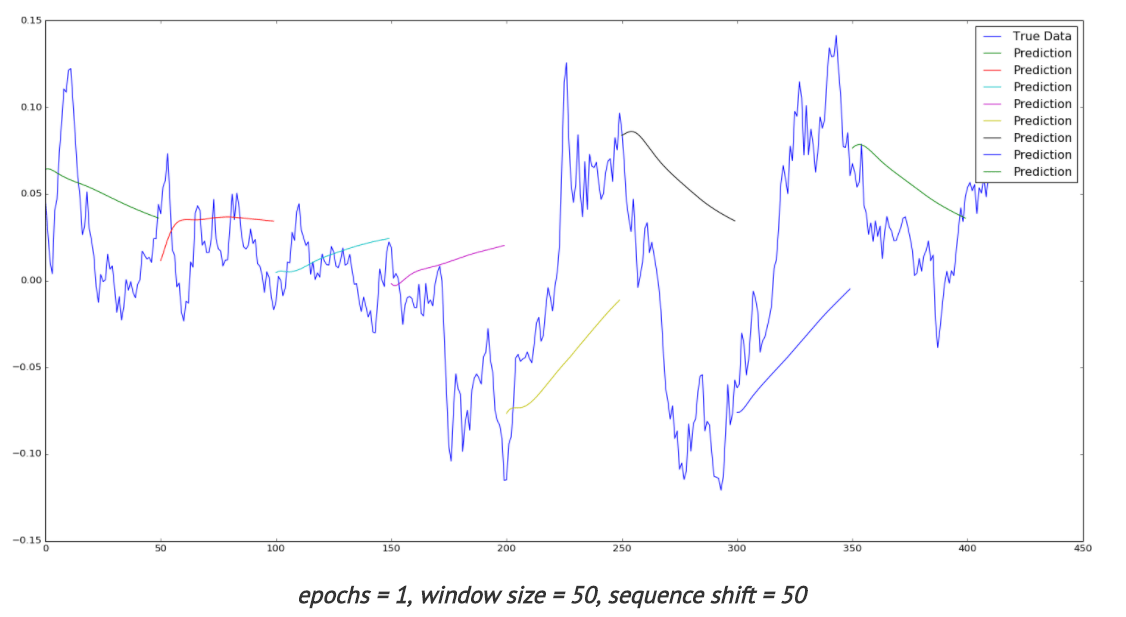Time series with LSTM

Below review is showing different approaches that can be used for forecasting time series data with convolutional neural networks.

1. Raw Data
The simplest way to feed data into neural network is to use raw data. Here is the link  to results of experiments with different types of neural networks including CNN. In this study stock data such as Date,Open,High,Low,Close,Volume,Adj Close were used with 3 types of networks: MLP, CNN and RNN.

CNN architecture was used as 2-layer convolutional neural network (combination of convolution and max-pooling layers) with one fully-connected layer. To improve performance the author suggests using different features (not only scaled time series) like some technical indicators, volume of sales.
According to  it is common to periodically insert a Pooling layer in-between successive Convolution layers in a CNN architecture. Its function is to progressively reduce the spatial size of the representation to reduce the amount of parameters and computation in the network, and hence to also control overfitting. The Pooling Layer operates independently on every depth slice of the input and resizes it spatially, using the MAX operation.

2. Automatic Selection of Features
Transforming data before inputting to neural network is common practice. We can use feature based methods like described here in Feature-selection-time-series-forecasting-python or filtering methods like removing trend, seasonality or low pass / high pass filtering.
With deep learning it is possible to lean features automatically. For example in one research the authors introduce a deep learning framework for multivariate time series classification: Multi-Channels Deep Convolutional Neural Networks (MCDCNN). Multivariate time series are separated into univariate ones and perform feature learning on each univariate series individually. Then a
normal MLP is concatenated at the end of feature learning to do classification. 
The CNN architecture consists of 2 layer CNN (combination of filter, activation and pooling layers) and 2 Fully connected layers that represent classification MLP.

3. Fully Convolutional Neural Network (FCN)
In this study different neural network architectures such as Multilayer Perceptrons, Fully convolutional NN (FCN), Residual Network are proposed. For FCN authors build the final networks by stacking three convolution blocks with the filter sizes {128, 256, 128} in each block. Unlike the MCNN and MC-CNN, any pooling operation is excluded. This strategy helps to prevent overfitting. Batch normalization is applied to speed up the convergence speed and help improve generalization.

After the convolution blocks, the features are fed into a global average pooling layer instead of a fully connected layer, which largely reduces the number of weights. The final label is produced by a softmax layer.  Thus the architecture of neural network consists of three convolution blocks and global average pooling and softmax layers in the end.

4. Different Data Transformations
In this study  CNNs were trained with different data transformations, which included: the entire dataset, spatial clustering, and PCA decomposition. Data was also fit to the hidden modules of a Clockwork Recurrent Neural Network. This type of recurrent network (CRNN) has the advantage of maintaining a high-temporal-resolution memory in its hidden layers after training.

This network also overcomes the problem of the vanishing gradient found in other RNNs by partitioning the neurons in its hidden layers as different ”sub-clocks” that are able to capture the input to the network at different time steps. Here you can find more about CRNN . According to this paper a clockwork RNN architecture is similar to a simple RNN with an input, output and hidden layer. The hidden layer is partitioned into g modules each with its own clock rate. Within each module the neurons are fully interconnected.

5. Analysing Multiple Time Series Relationships
This paper  focuses on analyzing multiple time series relationships such as correlations between them. Authors show that deep learning methods for time series processing are comparable to the other approaches and have wide opportunities for further improvement. Range of methods is discussed and code optimisations is applied for the convolutional neural network for the forecasting time series data domain.

6. Data Augmentation
In this study two approaches are proposed to artificially increase the size of training sets. The first one is based on data-augmentation techniques. The second one consists in mixing different training sets and learning the network in a semi-supervised way. The authors show that these two approaches improve the overall classification performance.

7. Encoding data as image
Another methodology for time series with convolutional neural networks that got popular with deep learning is encoding data as the image. Here data is encoded as images which feed to neural network. This enables the use of techniques from computer vision for classification.
Here  is the link where python script can be found for encoding data as image. It encodes data into formats such as GAF, MTF. The script has the dependencies on python modules such as Numpy, Pandas, Matplolib and Cpickle.

Theory of using image encoded data is described in . In this paper a novel framework proposes to encode time series data as different types of images, namely, Gramian Angular Fields (GAF) and Markov Transition Fields (MTF).

Learning Traffic as Images:
This paper  proposes a convolutional neural network (CNN)-based method that learns traffic as images and predicts large-scale, network-wide traffic speed with a high accuracy. Spatiotemporal traffic dynamics are converted to images describing the time and space relations of traffic flow via a two-dimensional time-space matrix. A CNN is applied to the image following two consecutive steps: abstract traffic feature extraction and network-wide traffic speed prediction. CNN architecture consists of several convolutional and pooling layers and fully connected layer in the end for prediction.

Conclusion

Deep learning and convolutional neural networks created new opportunities for forecasting time series data domain. The above text presents different techniques that can be used in time series prediction with convolutional neural networks. The common part that we can see in most of studies is that feature extraction can be done via deep learning automatically in CNN or in other words, CNNs can learn features on their own. Below you can see architecture of CNN at very high level. The actual implementations can vary in different ways, some of them were shown above.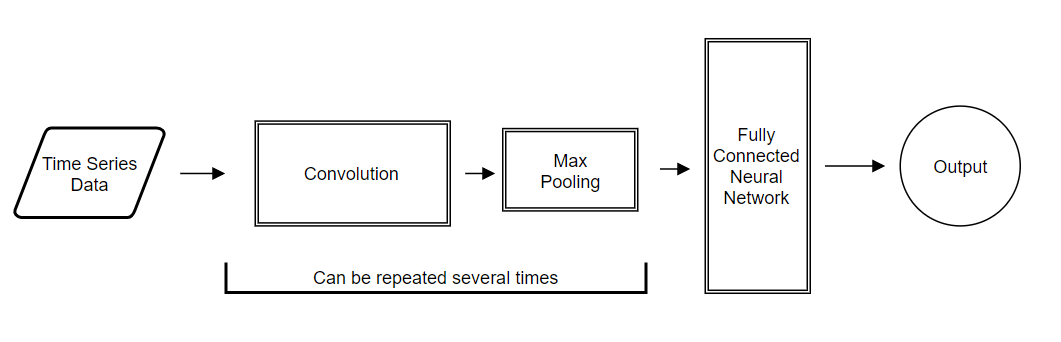CNN Architecture for Forecasting Time Series Data

References

## Converting Categorical Text Variable into Binary Variables

Sometimes we might need convert categorical feature into multiple binary features. Such situation emerged while I was implementing decision tree with independent categorical variable using python sklearn.tree for the post Building Decision Trees in Python – Handling Categorical Data and it turned out that a text independent variable is not supported.

One of solution would be binary encoding, also called one-hot-encoding when we might code [‘red’,’green’,’blue’] with 3 columns, one for each category, having 1 when the category match and 0 otherwise. 

Here we implement the python code that makes such binary encoding. The script looks at text data column and add numerical columns with values 0 or 1 to the original data. If category word exists in the column then it will be 1 in the column for this category, otherwise 0.

The list of categories is initialized in the beginning of the script. Additionally we initialize data source file, number of column with text data, and number of first empty column on right side. The script will add columns on right side starting from first empty column.

The next step in the script is to navigate through each row and do binary conversion and update data.

Below is some example of added binary columns to data input .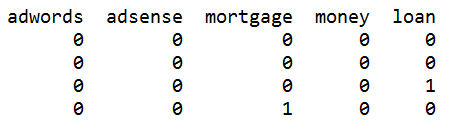Below is full source code.

``````
# -*- coding: utf-8 -*-

import pandas as pd

words = ["adwords", "adsense","mortgage","money","loan"]
data = pd.read_csv('adwords_data5.csv', sep= ',' , header = 0)

total_rows = len(data.index)

y_text_column_index=7
y_column_index=16

for index, w in enumerate(words):
data[w] = 0
col_index=data.columns.get_loc(w)

for x in range (total_rows):
if w in data.iloc[x,y_text_column_index] :
data.iloc[x,y_column_index+index]=1
else :
data.iloc[x,y_column_index+index]=0

print (data)
``````